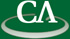## Strong Divisibility, Cyclotomic Polynomials, and Iterated Polynomials.

### Jeff Sommars

March 29th, 10:30am, K9509.

```Abstract:
In almost all algebra texts, one defines cyclotomic polynomials in
reference to the primitive n'th roots of unity. However, as I will show,
the property that gcd(x^n-1,x^m-1)=x^(gcd(m,n))-1 is sufficient to define
the cyclotomic polynomials uniquely as polynomials in Z[x].
As applications, I will consider implications for cyclotomic polynomials,
sequences of the form A^n-B^n, polynomial dynamical systems,
and rigid divisibility sequences.
```